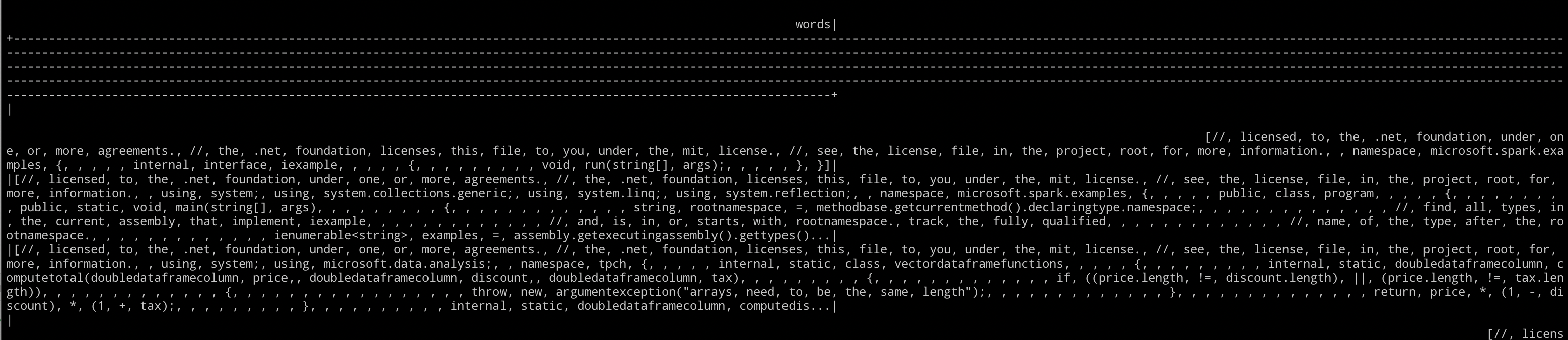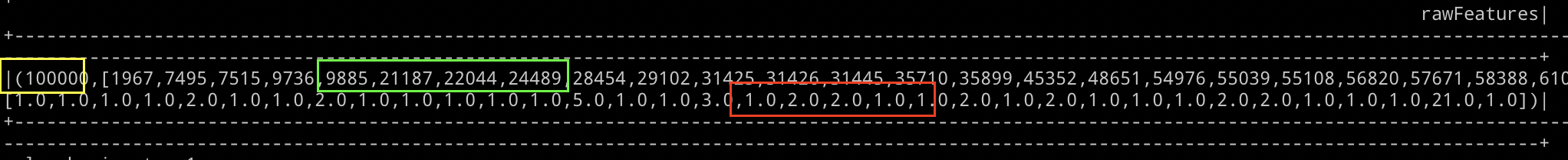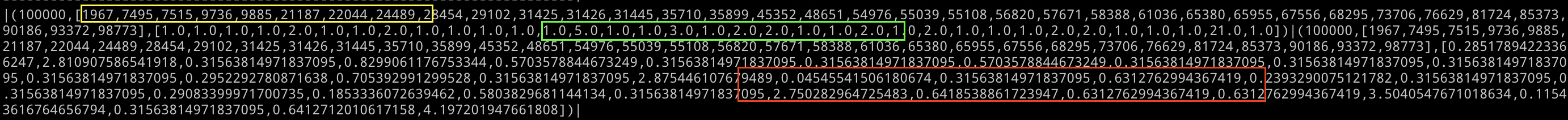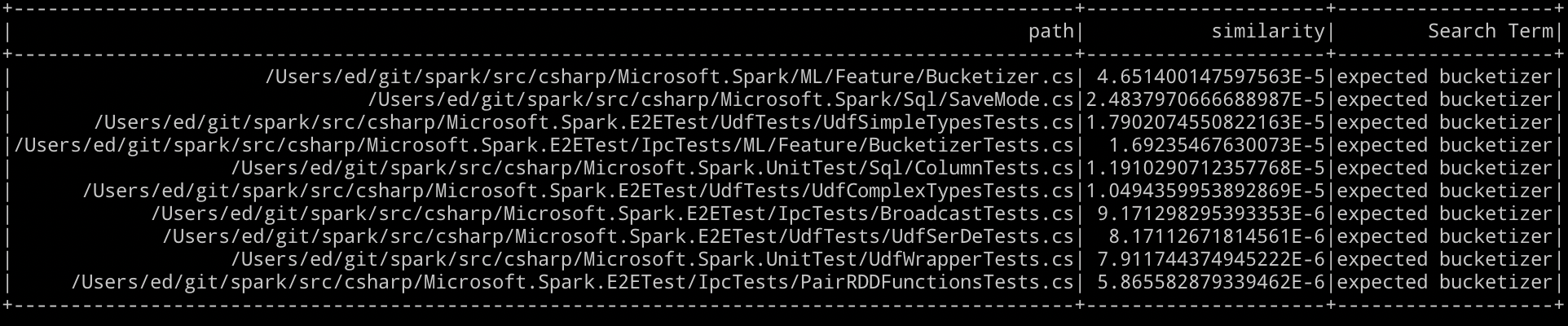# Spark ML in .NET for Apache Spark

Apache Spark has had a machine learning API for quite some time and this has been partially implemented in .NET for Apache Spark.

In this post we will look at how we can use the Apache Spark ML API from .NET. This is the second version of this post, the first version was written before version 1 of .NET for Apache Spark and there was a vital piece of the implementation missing which meant although we could build the model in .NET, we couldn't actually use it. The necessary functionality is now available and so I am updating the post. To see the previous version go to: https://the.agilesql.club/2020/07/tf-idf-in-.net-for-apache-spark-using-spark-ml/

## What can you do in Spark for Machine Learning?

In native Apache Spark, the first Machine Learning API was called MLLib, this used RDD's and so when the DataFrame API was released, a second API was also released the Spark ML API. There was some inconsistency with how the API's were named but most of the newer objects were available in the Spark.ML namespace so we call it Spark.ML as opposed to MLLib, which is the RDD version of the API's and not supported in .NET for Apache Spark.

At the end of last year, .NET for Apache Spark had no support for either the Spark.ML or Spark.MLLib API's so I started implementing Spark.ML. In a similar way that .NET for Apache Spark supports the DataFrame API and not the RDD API, I started with Spark.ML and I believe that having the full Spark ML API will be enough for anyone.

The Spark ML API is broadly broken up into:

• Basic statistics
• Data sources
• Extracting, transforming and selecting features
• Classification and Regression
• Clustering
• Collaborative filtering
• Frequent pattern mining
• Model selection and tuning

## Where are we now?

Well, a few months on and a few of the objects have been implemented, there is a long way to go to complete the Spark ML API, but it is a start (if you are interested in helping to implement the API (or any of the API's) and you need help then feel free to reach out and ask and I will try to help).

The link above (https://spark.apache.org/docs/3.0.0/ml-guide.html) is a great place to start with ML in Spark. There are lots of examples of how to make useful things.

## Implemented objects

Microsoft.Spark.ML.Feature:

• Bucketizer
• HashingTF
• IDF
• IDFModel
• Tokenizer
• Word2Vec
• Word2VecModel
• CountVectorizer
• CountVectorizerModel
• FeatureHasher
• StopWordsRemover

If we go back to that article I mentioned earlier and the features section: https://spark.apache.org/docs/3.0.0/ml-features.html what we start with is the TF-IDF sample. TF-IDF (see https://en.wikipedia.org/wiki/Tf%E2%80%93idf) is a way to find relevant documents in a set of documents. First the technical description: “Term frequency-inverse document frequency (TF-IDF) is a feature vectorization method widely used in text mining to reflect the importance of a term to a document in the corpus” which roughly translates to “Find documents that include this term but only if the term appears lots of times in the document relative to the size of all the documents” so if we had these two documents:

“My favourite thing to do is to go for a stroll. The key to a good stroll in the park is to be able to walk in peace.”

“When I was little I used to like to run in the park, the most important thing about running in the park is that it really invigorates you and makes you feel alive. When I haven't been able to run I try to at least go for a stroll, yes a good stroll but nothing beats a good run.”

The first document is all about going for a stroll, that is the key concept. The second document mentions going for a stroll, but the fundamental concept is running. The idea of TF-IDF is that even if both documents mention the same word twice, the importance of it in the second document is less because the second document specifies other words more times.

## So how do we implement this in Spark?

First of all, we take a set of documents, then we build a model by:

• Splitting each document into a series of words using Tokenizer
• Use HashingTF to convert the series of words into a Vector that contains a hash of the word and how many times that word appears in the document
• Create an IDF model which adjusts how important a word is within a document compared to all words in all the documents, so run is important in the second document but stroll less important

This creates a model which we can then use to search for documents matching our term.

## And we can do all this with just what has been implemented already?

YES! There was a time pre .NET for Apache Spark 1.0 where we could build the model in C# but then needed to switch to scala to use a vector from a UDF.

### Prerequisites

To go through this demo you will need .NET core and the .NET for Apache Spark nuget package as well as a working Apache Spark installation. I would recommend, at a minumum, you get this tutorial running: https://dotnet.microsoft.com/learn/data/spark-tutorial/intro

You will also need to use .NET for Apache Spark 1.0 or higher and I would recommend at least Apache Spark 3.0 (older versions will work)

I have put a full copy of the demo:

https://github.com/GoEddie/Spark-Dotnet-TF-IDF

We are going to walk through an example of reading a load of source code files, converting the documents to a DataFrame, train a model on the documents and then querying the model to find out the most relevant documents. I am going to use the .NET for Apache Spark repo (https://github.com/dotnet/spark) as my data source. The goal is to be able to find relevent code files in the repo.

### 1. Create a new console app and add a reference to Microsoft.Spark

``````using System;
using System.Collections;
using System.Collections.Generic;
using System.IO;
using Microsoft.Spark.ML.Feature;
using Microsoft.Spark.Sql;
using Microsoft.Spark.Sql.Types;
using static Microsoft.Spark.Sql.Functions;
``````

Note here that we have a `using static Microsoft.Spark.Sql.Functions`, which means we can use the methods on the object directly such as `Col`, the alternative is to reference each method using the object such as `Functions.Col` but I prefer the first approach.

### 3. Handle some args

We are going to want to pass in an argument for the source folder and the the search term to match documents against.

``````namespace sparky
{
internal class Program
{
private static void Main(string[] args)
{
if (args.Length != 2)
{
Console.WriteLine("Args!");
return;
}

var sourceDir = args;
var searchTerm = args;
``````

### 4. Create a spark session

``````var spark = SparkSession
.Builder()
.GetOrCreate();
``````

### 5. Create an object to store documents

``````private class Document
{
public string Content;
public string Path;
}
``````

### 6. Create a method to gather our documents

``````private static List<Document> GetSourceFiles(string gitSpark)
{
var documents = new List<Document>();

foreach (var file in new DirectoryInfo(gitSpark).EnumerateFiles("*.cs", SearchOption.AllDirectories))
{
Path = file.FullName,
});

return documents;
}
``````

### 7. Take the list of documents and convert to a DataFrame

``````static DataFrame toDF(List<Document> docs){

var rows = new List<GenericRow>();

var spark = SparkSession.Active();

foreach(var row in docs){
}

var schema = new StructType(new List<StructField>()
{
new StructField("Path", new StringType()),
new StructField("Content", new StringType())
});

return spark.CreateDataFrame(rows, schema);
}
``````

### 8. Now the interesting bit, split the documents into words

``````var tokenizer = new Tokenizer();
var words = tokenizer
.SetInputCol("Content")
.SetOutputCol("words")
.Transform(sourceDocuments);
``````

This pattern of create an object (Tokenizer) then call SetInputCol and SetOutputCol are used across the Spark.ML objects so get used to it. Also, note that a call to a method like SetInputCol returns a new object so you can't do something like:

``````tokenizer.SetInputCol(str)
tokenizer.SetOuputCol(str)
``````

If you do this, then you won't be changing your original tokenizer.

`words` in this context is a DataFrame that contains a column called “words” which contains each document as an array of words:### 9. Convert words into vectors

Computers are awesome, but they don't understand words very well, a hippopotamus is a massive animal but all a computer understands is that is there is a memory address with a series of bytes, it doesn't even know the difference between a pair of pants (English) and a pair of pants (American). Computers are however very good with numbers so we will use a `HasingTF` to convert our array of individual words into a set of numbers:

``````var hashingTF = new HashingTF()
.SetInputCol("words")
.SetOutputCol("rawFeatures")
.SetNumFeatures(100000);

var featurizedData = hashingTF.Transform(words);
``````The vector contains the number of words we have in each row (yellow), each word hashed into a number (green) and the number of times each word was found (red).

### 10. Fit our data to an IDF model

The IDF model takes our terms and works out for each document what the relevance of each term is in relation to the whole document, to do this we “Fit” the features, which means to train the features to create our model i.e. we take the words and documents and entire text across all of the documents and create a model that includes the term frequencies and the weight of each term relevent to the document.

``````var idf = new IDF().SetInputCol("rawFeatures").SetOutputCol("features");
var idfModel = idf.Fit(featurizedData);
var filtered = rescaled.Select("Path", "features");
``````

This means we now have the terms (yellow), the frequency of each term (green) and now the weighted importance of the term relative to the size of the document weighted by how often the word appears in all the documents(red):Later, we will calculate the cosine similarity of each document and of our search terms, to calculate this we will need to normalize each vector. What we do is multiple each item in the vectors attributes and then take the square root:

``````private static readonly Func<Column, Column> udfCalcNorm = Udf<Row, double>(row =>
{
var values = (ArrayList) row.Values;
var norm = 0.0;

foreach (var value in values)
{
var d = (double) value;
norm += d * d;
}

return Math.Sqrt(norm);
}
);
``````

### 11. Save our work

If we were creating a model of a large set of documents and then searching later we could choose to save the model and then when we want to query the model, load the model in. To save the model and the other objects we could do this:

``````tokenizer.Save(Path.Join(tempDir, "temp.tokenizer"));
hashingTF.Save(Path.Join(tempDir, "temp.hashingTF"));
filtered.Write().Mode("overwrite").Parquet(Path.Join(tempDir, "temp.parquet"));
idfModel.Save(Path.Join(tempDir, "temp.idfModel"));
``````

### 12. Generate vector for search terms

Because we only have a small model, we can build it at runtime so we then need to create a DataFrame that contains the search term and everything we need to be able to compare each document in our model.

``````private static DataFrame GetSearchTermTFIDF(SparkSession spark, string searchTerm, Tokenizer tokenizer,HashingTF hashingTF, IDFModel idfModel)
{
var searchTermDataFrame = spark.CreateDataFrame(new List<string>() {searchTerm}).WithColumnRenamed("_1", "Content");
var searchWords = tokenizer.Transform(searchTermDataFrame);
var featurizedSeachTerm = hashingTF.Transform(searchWords);
var search = idfModel.Transform(featurizedSeachTerm).WithColumnRenamed("features", "features2").WithColumn("norm2", udfCalcNorm(Col("features2")));
return search;
}
``````

Now we have our original documents and our search term in a similar format, they both have a vector and the normalized vector, if we join them together, we can use spark to calculate the cosine similarity:

``````var results = searchTermTfIdf.CrossJoin(normalized);

results
.WithColumn("similarity",udfCosineSimilarity(Col("features"), Col("features2"), Col("norm"), Col("norm2")))
.Select("path", "similarity")
.Filter("similarity > 0.0")
.OrderBy(Desc("similarity"))
.Limit(10)
.WithColumn("Search Term", Lit(searchTerm))
.Show(10, 100000);
``````

We define the UDF for calculating the cosing similarity:

``````private static readonly Func<Column, Column, Column, Column, Column> udfCosineSimilarity =
Udf<Row, Row, double, double, double>(
(vectorA, vectorB, normA, normB) =>
{
var indicesA = (ArrayList) vectorA.Values;
var valuesA = (ArrayList) vectorA.Values;

var indicesB = (ArrayList) vectorB.Values;
var valuesB = (ArrayList) vectorB.Values;

var dotProduct = 0.0;

for (var i = 0; i < indicesA.Count; i++)
{
var valA = (double) valuesA[i];

var indexB = findIndex(indicesB, 0, (int) indicesA[i]);

double valB = 0;
if (indexB != -1)
{
valB = (double) valuesB[indexB];
}
else
{
valB = 0;
}

dotProduct += valA * valB;
}

var divisor = normA * normB;

return divisor == 0 ? 0 : dotProduct / divisor;
});
``````

There is something here I want to point out:

``````
var indicesA = (ArrayList) vectorA.Values;
var valuesA = (ArrayList) vectorA.Values;

var indicesB = (ArrayList) vectorB.Values;
var valuesB = (ArrayList) vectorB.Values;

var indexB = findIndex(indicesB, 0, (int) indicesA[i]);

private static int findIndex(ArrayList list, int currentIndex, int wantedValue)
{
for (var i = currentIndex; i < list.Count; i++)
if ((int) list[i] == wantedValue)
return i;

return -1;
}
``````

The UDF receives a Row of values, two values are ArrayLists which make up a single SparseVector. The point of a SparseVector is to save space where a value is 0, it doesn't need to be stored in the array. A SparseVector is defined as an array of: TypeOfVector (SparseVector = 0, DenseVector = 1), LengthOfVector, Index, Values. A Sparse Vector skips out any values which are equal to 0 so if you wanted to express this vector as a sparse vector it would go from:

``````1, 2, 3, 0, 0, 0, 0, 0, 4, 0, 0
``````

to:

``````1,2,3,4
``````

and we use the indices to see which values are set:

``````0,1,2,8
``````

So our sparse vector will look like:

``````0, 11, [0,1,2,8], [1,2,3,4]
``````

To see what value is at offset 0 in the array you lookup 0 in the indices and as it is at position 0 you look in the values array at position 0. If you wanted to know what the 6th value was in the array you would look in the indices for the number 5 (0-th) and as it doesn't exist you know that it is actually 0.

If you conside that a SparseVector might contain many values, removing any zero makes sense in some cases.

Now onto the cosine similarity, which we get by multiplying each item in the vector one by one, then dividing that value by the normalized value we already worked out - remember the vector we have is a sparse vector so we first need to use the indices to lookup the value (or realise that it is 0)

So in Pseudo code:

``````For each item in the vector:
Multiply each value from each document against each value in our search term
Divided by the result of multiplying the square root of each of the vectors total of each length and direction
``````

This then gives us a way to order the results by closest match first, if we run this and search for “expected bucketizer” we should see something similar to:Which looks like a pretty good match to me, “Bucketizer.cs” is relevant for bucketizer and expected is in “SaveMode.cs” and as it is a small document, the meaning of expected is important. “UdfSimpleTypesTests.cs” has expected 12 times - still, it is a much bigger document, so I am going to say that the term frequency compared to the inverse document frequency (TF-IDF) works pretty well using .NET for Apache Spark.

I hope you have found this useful. The full repo is available:

https://github.com/GoEddie/Spark-Dotnet-TF-IDF